In our previous courses, we have seen how to perform the four fundamental operations (add, subtract, multiply and divide) on both natural numbers and integers. However, we didn’t handle more complex cases such as when it was not dividing things up exactly. This is the subject of this important course: the Euclidean division — or division with remainder.

Don’t worry, the name and importance hide something very easy to understand and perform ;)

Euclidean division, and algorithms to compute it, are fundamental for many questions such as for finding the greatest common divisor (GCD) or in modular arithmetic - modulo operation (used absolutely everywhere in computer science: from website to cryptography through video games).

But first, a small reminder about the division!

The division allows us to calculate the sharing problems, it is the opposite of multiplying.

Example:
There are 12 blue candies, and 4 friends want to share them, how do we divide the candies?

The answer may seem evident: if each friend takes 3 candies, we are all good. Division is splitting into equal parts or groups. It is the result of "fair sharing".

Note:
As we said, another way to think of division is as the opposite of multiplication. Taking this example we got:

--> 12 / 4 = 3 (we gives 3 candies to each of us)

Now if we invert the operation using the result, we replace the ‘=’ with a ‘x’ sign and the ‘/’ with an ‘=’ sign:

--> 12 = 3 x 4 (if 3 candies are given to each of us, we shared a total of 12 candies)

This is, indeed, the mathematical definition of the division: $$\pmb{a / b = a * \frac{1}{b}}$$
Using multiplication is a great way to check our division and master our math tests. But remember this rule, it is the only one to know when dealing with division:
We never divide by 0!

### But... Sometimes it does not fit perfectly!

Let's deal with it!

As we have seen, division is breaking a number up into an equal number of parts, but... there may be something left over. Let’s go back on our previous example, however, this time the 4 friends have 13 (one more than before) blue candies to share:

We can see that there is 1 left over; we call that the Remainder.
Note: it may eventually be possible to cut the remaining candy in four (a quarter - 1/4 - of candy for each), but this is addressed in the course dealing with Fractions.
We will now learn how to perform all divisions (even with large numbers) by hand. But first, a quick overview of the vocabulary.

## Dividend, Divisor, Quotient and Remainder

Each part of a division has a name:

- Dividend: The dividend is the number you are dividing up.
- Divisor: The divisor is the number you are dividing by.
- Quotient: The quotient is the main result (the number of candies you can give to each one).
- Remainder: The left over.

For instance: 13 (dividend) ÷ 4 (divisor) = 3 (quotient) 'R'1 (remainder)

Great, I think we are now fully ready to tackle the process!

To perform a division by hand, every student learns (without knowing) an algorithm which is one of the oldest algorithms in use (it appeared in Euclid's Elements around 300 BCE).

We will decompose the process step-by-step (only 2 steps to repeat) through an example: 533 ÷ 4.

Here is the color legend used:

Red: the number we will try to divide by our divisor.
Blue: the divisor, how many of them do we have within the red number?
Green: the amount of divisor found within the red number.
Yellow: subtracting ‘Blue x Green’ from the red number.

#### Starting point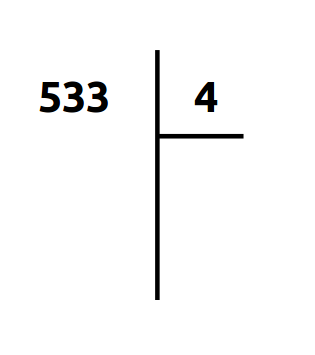We start the process using the most left digit of the dividend.

#### A. How many times do we have 4 (blue) in 5 (red)?In five, we may count to four only 1 time (green).

We put this number in the quotient box (green) and perform the subtraction (yellow):
5 - (4 x 1) = 1

#### B. Bring down the next digits of the dividend.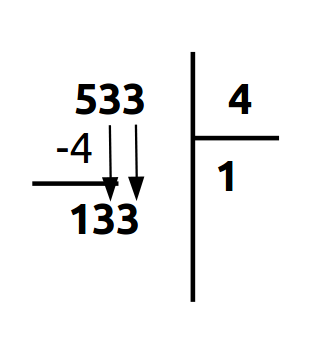All we have to do now is to bring down the next digits of the dividend and repeat from A.

Now, we just repeat with the process with the result of the subtraction as the dividend.

#### A. How many times do we have 4 (blue) in 1 (red)?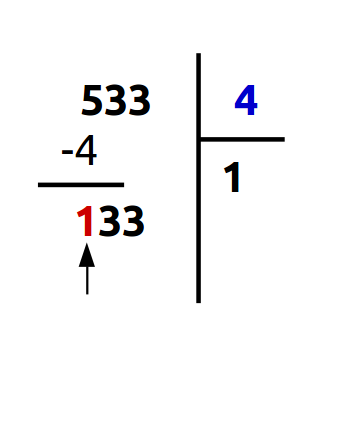Here, 1 is less than 4. In this case, all we have to do is repeating this step including the next digit of the dividend (cf. next).

#### A.bis Include the next digit of the dividend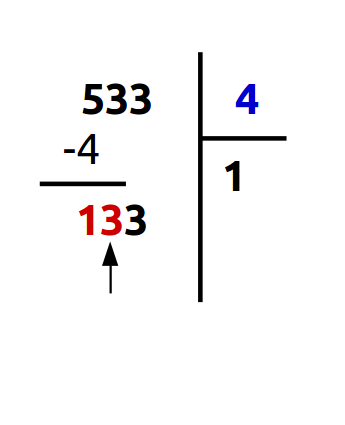As we said, we include the next digit of the new dividend (red) to perform this step.

#### A. How many times do we have 4 (blue) in 13 (red)?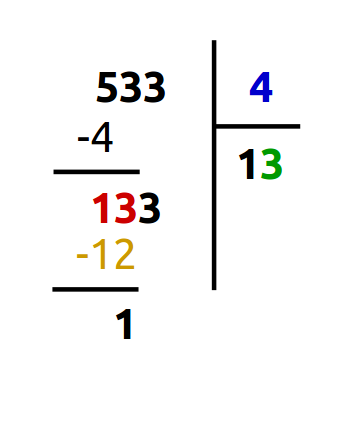In thirteen, we may count to four only 3 times (green).

We put this number in the quotient box (green) and perform the subtraction (yellow):
13 - (4 x 3) = 1

And finally...

#### B. Bring down the next digits of the dividend.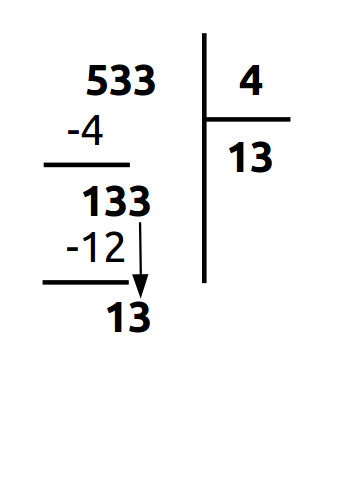Just like before (step B), we bring down the next digits of the new dividend and repeat from A.

#### A. Finished!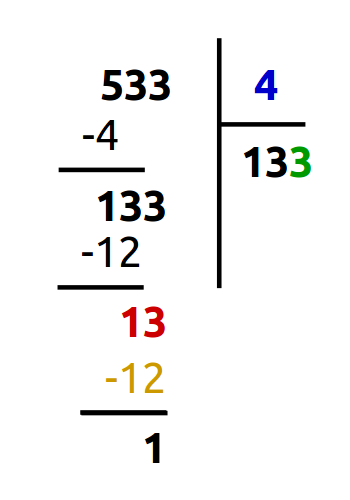Here we perform the same operations as before...

Once the new dividend is less than the divisor, our process is over!

We found out that: 533 ÷ 4 = 133, 'R'1
We can also say that: 533 = 4 x 133 + 1

Woaw, this is very handy!

To always find the GCD we will use Euclid's algorithm:

- Perform the Euclidean division of the greatest number (noted a) of the fraction on the smallest number (noted b) and keep the rest (noted r).

- As long as the rest is different from 0, we reiterate the division replacing a by b and b by r.

We will then be able to write: $$1053 = 81 \color{green}{\textbf{ * 13}}$$ $$325 = 25 \color{green}{\textbf{ * 13}}$$

We will use the GCD a lot starting with mastering the fractions whether to simplify them or to perform operations.